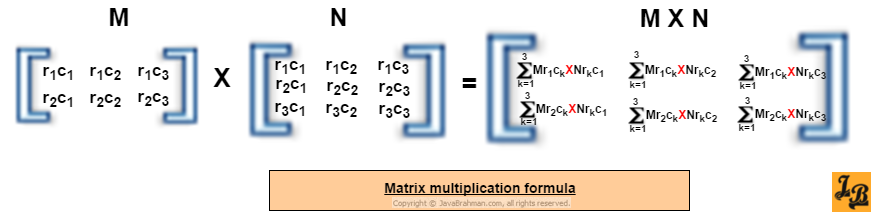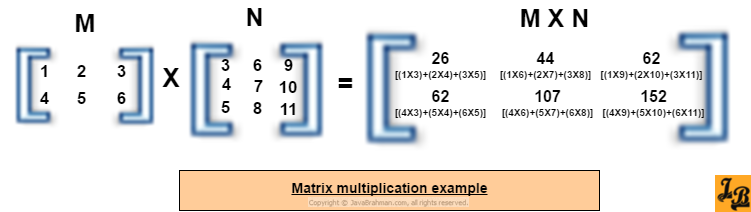Java program for matrix multiplication of dynamically sized matrices
This tutorial first explains how to perform matrix multiplication. It then shows how to implement matrix multiplication in java and explains the java code in detail. How to perform matrix multiplication Matrix multiplication formula-
Given two matrices, M and N, with the number of columns of M being equal to number of rows of N, the product of these matrices, denoted by 'MXN', contains consists of elements calculated using the formula -
MXN(ricj) = M(rick X N rkcj)
Where,
- MXN(ricj) is the number in ricj position of MXN.
- the value of k varies from 1 to 'no. of columns in M'.
The multiplication formula in matrix form looks like this -An example of matrix multiplication would then look like this -Having understood how matrix multiplication is done, let us now see the java program for matrix multiplication, followed by the explanation of the code.
Matrix multiplication program in Java
Java program to multiply 2 matrices
``````package com.javabrahman.generaljava;
public class MatrixMultiplication {
public static void main(String args[]) {
int m1[][] = {{1, 2, 3},
{4, 5, 6}};
int m2[][] = {{3, 6, 9},
{4, 7, 10},
{5, 8, 11}};
int product[][] = multiplyMatrices(m1, m2);
if (product != null) {
printMatrix(m1);
System.out.println(" X ");
printMatrix(m2);
System.out.println(" = ");
printMatrix(product);
}
}

/**
* Method to print a 2-D matrix row-wise
*
* @param matrix
*/
public static void printMatrix(int[][] matrix) {
for (int i = 0; i < matrix.length; i++) {
for (int j = 0; j < matrix.length; j++) {
System.out.print(" " + matrix[i][j]);
}
System.out.println();
}
}

/**
* Method to multiply matrices
* @param matrix1
* @param matrix2
*/
public static int[][] multiplyMatrices(int[][] matrix1, int[][] matrix2) {
int m1_rows=matrix1.length;
int m1_columns=matrix1.length;
int m2_rows=matrix2.length;
int m2_columns=matrix2.length;
int[][] product = new int[m1_rows][m2_columns];
if ((m1_columns != m2_rows)) {
System.out.println("Error - Number of columns of matrix1 IS NOT EQUAL TO number of rows of matrix2.");
} else {

for (int i = 0; i < m1_rows; i++) {
for (int j = 0; j < m2_columns; j++) {
for(int k=0;k < m1_columns;k++){
product[i][j]+=matrix1[i][k]*matrix2[k][j];
}
}
}
}
return product;
}
}``````
OUTPUT of the above code
```1 2 3
4  5  6
X
3  6  9
4  7  10
5  8  11
=
26   44   62
62  107  152```
Explanation of the code
• `MatrixMultiplication` is class in which static method `multiplyMatrices()` method contains the implementation for multiplying 2 matrices.
• `printMatrix()` method simply prints the matrix in a pretty format as rows and columns.
• In the `main()` method, first 2 matrices - `m1` and `m2` - are defined as 2-dimensional `int[][]` arrays. The arrays are initialized during definition with `m1` having 2 rows and 3 columns, and `m2` having 3 rows and 3 columns.
• `m1` and `m2` are then sent as parameters to `multiplyMatrices()` method, where the matrices are received into parameters named `matrix1` and `matrix2` respectively.
• In `multiplyMatrices()` - first the mandatory condition for matrix multiplication is checked. I.e. whether matrix1's number of columns is same as matrix2's number of rows. If not - an error is thrown and matrix multiplication is skipped with a `null` value being returned.
• The actual logic for matrix multiplication is inside 3 nested loops. The outermost loop is for rows, the middle loop is for columns and the innermost loop sums together the products of correponding row and column elements as explained via the matrix multiplication formula and example above.
• The matrix `product`, which is a matrix of 2 rows and 3 colums is sent back as the return value to the `main()` method where it is printed, using `printMatrix()` method, along with the two matrices which were multiplied, in the M X N = MN format.